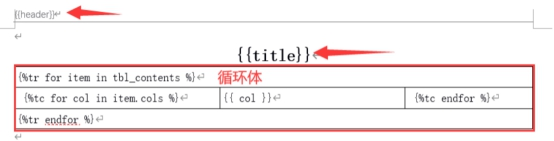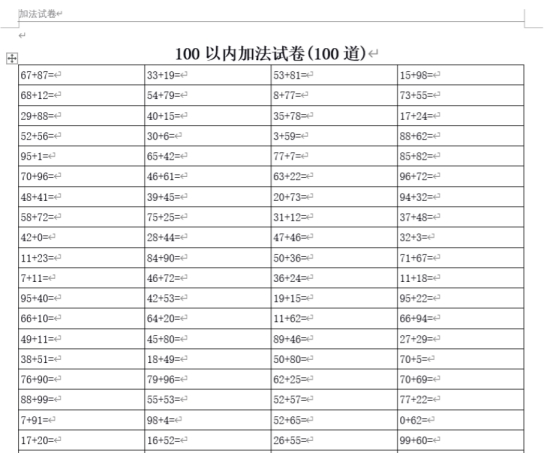# Python如何自动生成考试试卷？1、模板文件2、实现代码

```from docxtpl import DocxTemplate
import os
import random
import pymysql
cur_path = os.path.dirname(__file__)
tempfilename = os.path.join(cur_path, 'template', '加法模板.docx')
savefilename = os.path.join(cur_path, '加法.docx')
tpl = DocxTemplate(tempfilename)

list1 = []
def build_data():
for x in range(25):
items = {}
list2 = []
for y in range(4):
num1 = random.randint(0,99)
num2 = random.randint(0,99)
list2.append(f'{num1}+{num2}=')
items.setdefault('cols', list2)
list1.append(items)

context = {
'title': '100以内加法试卷(100道)',
'tbl_contents': list1,
'footer': '2020-09-13',
}
tpl.render(context)
tpl.save(savefilename)

if __name__ == "__main__":
build_data()```